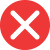simpler

Correct spelling, explanation: simpler is the correct spelling because it is a comparative form of the adjective simple. One way to create a comparative adjective is to add -er ending to the base form, or only -r in case of words ending with e. Simple ends with e so we add only -r, hence simpler. Simplier is therefore incorrect.

Definition of simpler:
adjective, comparative form from simple, easier, less complicated
Last week’s test was much simpler than today’s one!
Don’t you think taxes should be simpler?simplier

Incorrect spelling, explanation: simplier is incorrect because to create a comparative adjective we need to add -r, -er, or the word more to the base form. In the case of simple, we add -r because the base form already ends with e. Therefore simpler is the correct form. Simplier is a mistake and might be made due to the adverbial form simply – it might have been mistaken with an adverb and thus with the words ending with y, this consonant would transform to i when adding the suffix. Here however we need an adjective base – so simpler is the correct form.